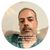# QlikView App Dev

Discussion Board for collaboration related to QlikView App Development.

Announcements
Support Cases coming to Qlik Community Oct. 4! Start chats, open cases, explore resources: READ DETAILS
cancel
Showing results for
Did you mean:Not applicable

## 3 Year Rolling Average

I have used an expression to figure out the 3 year rolling average, but how do i show only the most recent for example. I only want to show the most recent rolling 3 year average so i only want to show the value for 2014, but when i try to hide the other years it effects the expression so the value is not the same. Any ideas?

Year      Rolling

2011       2906

2012       1732

2013       1876

2014       1036

1 Solution

Accepted SolutionsCreator II

hi Zeth,

if your condition is when no selection is made rolling 3 yr. calculation should be shown and if any selection is done on year then only that year's value should be show up then use the below expression.

=if(Only(Year1),sum ({<Year1={'=\$(=max(Year1))'}>}Rolling),

sum ({<Year1={'>=\$(=max(Year1)-2)<=\$(=max(Year1))'}>}Rolling))

3 RepliesMVP

It would be much easier to explain if you posted a sample.MVP

Did you use the expression like this for rolling?

sum(aggr(rangesum(above(total sum({<Year=>}Amount),0,3)),Year))

To see the latest year, you may need to try set exp like below-

{<Year={\$(=Max(Year))}

Or follow the post Calculating rolling n-period totals, averages or other aggregations‌ for detailed understanding

posted by  gwassenaar

Always share sample data for faster response as troyansky suggested. Thanks.Creator II

hi Zeth,

if your condition is when no selection is made rolling 3 yr. calculation should be shown and if any selection is done on year then only that year's value should be show up then use the below expression.

=if(Only(Year1),sum ({<Year1={'=\$(=max(Year1))'}>}Rolling),

sum ({<Year1={'>=\$(=max(Year1)-2)<=\$(=max(Year1))'}>}Rolling))Community Browser